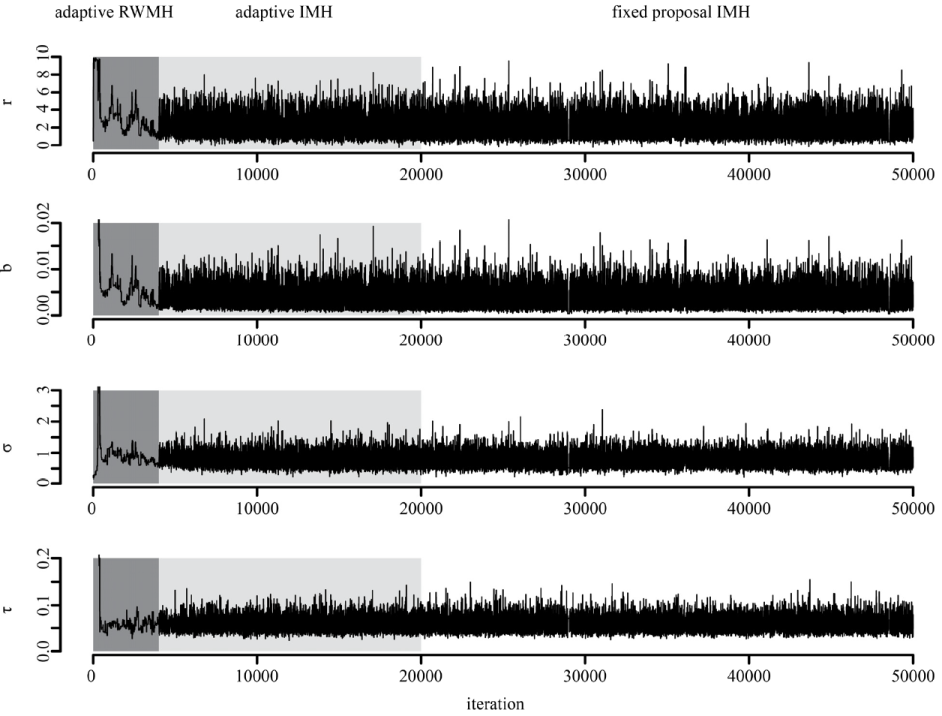### Jonas Knape and Perry de Valpine. 2012. Fitting complex population models by combining particle filters with Markov chain Monte Carlo. Ecology 93:256–263.

Appendix A. An adaptive Metropolis-Hastings scheme.

The adaptive Metropolis-Hastings (MH) scheme was divided into three parts, (i) an adaptive random-walk MH phase for initial exploration of the posterior distribution, (ii) an adaptive independence MH phase for fine tuning proposals and (iii) a fixed proposal independence MH whose output was used for analysis. The two first phases are a modified version of the scheme given by Pitt et al. (2010). Below we will assume that the dimension of the parameter space is d, i.e. d is the number of parameters over which the particle MCMC algorithm is run.

Random-walk phase

For the random-walk phase we used multivariate random-walk proposals, θ(i)* = θ(i-1) + εi where εi is drawn from a zero mean multivariate normal mixture distribution. From iteration 1 and until 2d Metropolis Hastings proposals had been accepted, εi was drawn from the normal distribution N(0, 0.1Id) where Id is the identity matrix. Thereafter a proposal was constructed at each iteration from the sample covariance matrix Si of the history of the MCMC chain, from i.e. the vectors θ(1), θ(2),…, θ(i-1). Specifically, the proposal at iteration i was drawn from the mixture εi ~ 0.4 N(0, 0.1Id) + 0.5 N(0, 2.382 / d Si) + 0.1 N(0, 5·2.382 / d Si). The first component allows component-wise independent and un-scaled moves. The second component adapts its variance according to the variance of the chain using the scaling 2.382 / d as suggested by Roberts and Rosenthal  (2009). The third component is an inflated version of component 2 allowing for larger jumps in parameter space.

After 4000 iterations the random-walk proposals were abandoned and replaced by independence proposals θ(i)* = θi where θi is drawn from multivariate normal mixture. The underlying idea is that after the random-walk phase a sufficiently good picture of the posterior should have been obtained in order to construct a normal mixture that at least crudely covers and approximates the high density regions of the posterior. The approximation is built from the history of the chain using the k-means clustering algorithm. This is a heuristic algorithm that given a sequence of multivariate data points and a fixed number of clusters, k, partitions the data points into k classes in order to minimize the within cluster sum of squares. We first linearly transformed the MCMC chain to obtain equal sample variances for all components. For k = 1, …, 6, we applied the k-means algorithm to the transformed sample (using the R function kmeans). Using the resulting classifications for each k, a multivariate normal distribution was fitted to the original MCMC values within each of the k clusters (using the sample mean and sample covariance matrix). The BIC of the multivariate normal mixture with the fitted components and weights equal to the proportion of points within each of the classes (as computed by k-means) was then computed for each k. Finally, the mixture proposal was constructed by combining the mixture with the lowest BIC selected from the above with another mixture having the same weights, means and number of components but with the component variances inflated by a factor 5. The inflated components serve as a safe guard in case the high density regions of the posterior have not yet been explored by the chain.

After in total 20000 iterations the adaptations of the independence proposals were stopped and the last proposal mixture was used for 30000 iterations.FIG. A1. Trace plots of the particle MCMC for the logistic diffusion model. The first 4000 iterations constitute an adaptive random-walk Metropolis-Hastings phase (dark shading), the next 16000 iterations an adaptive independence Metropolis-Hastings phase (light shading) and the final 30000 iterations an independence Metropolis-Hastings phase with a fixed proposal distribution.

LITERATURE CITED

Pitt, M.  Silva, R., Giordani, P. and R. Kohn. 2010. Auxiliary particle filtering within adaptive Metropolis Hastings sampling. Preprint arXiv:1006.1914v1.

Roberts, G. O., and J. S. Rosenthal. 2009. Examples of Adaptive MCMC. Journal of Computational and Graphical Statistics 18:349–367.

[Back to E093-025]